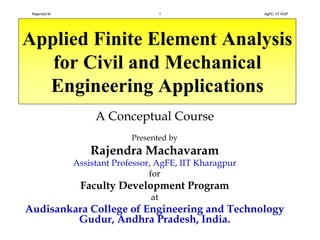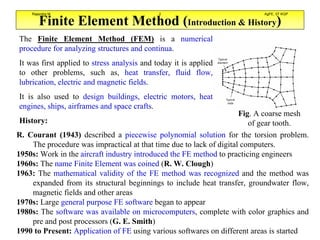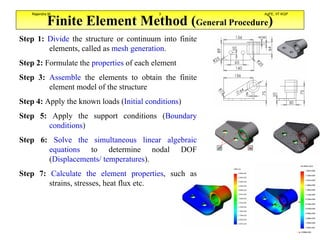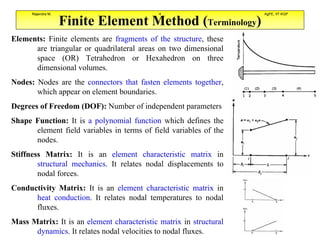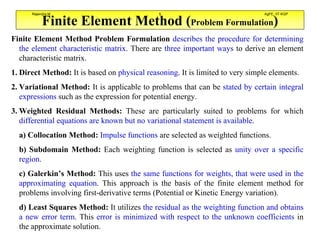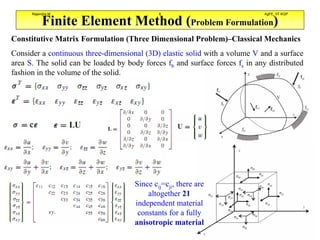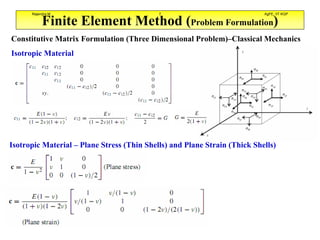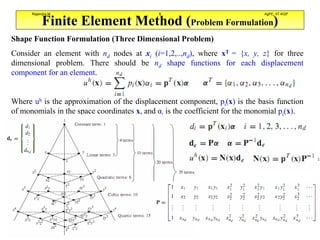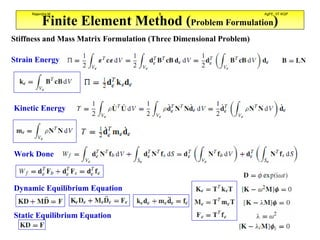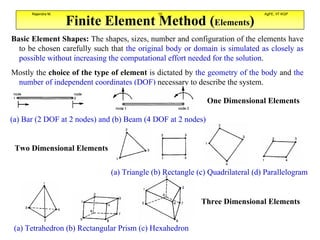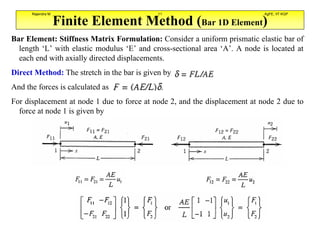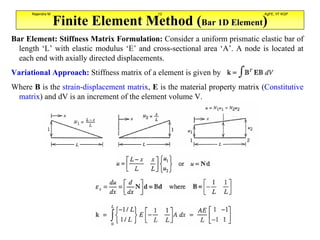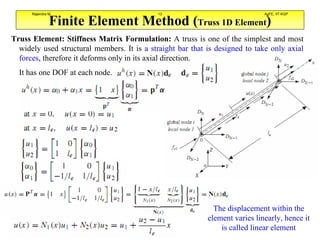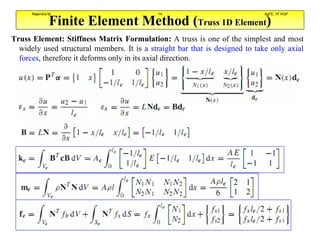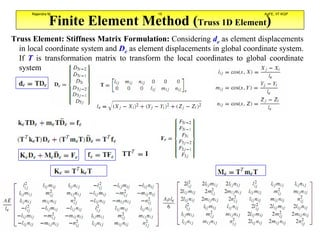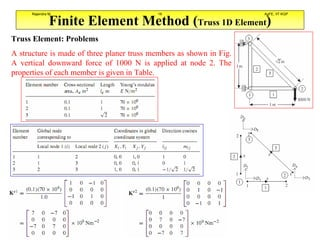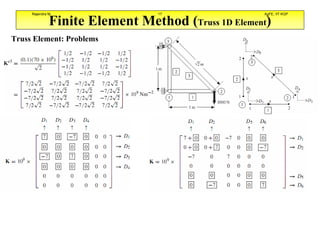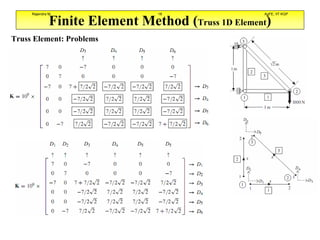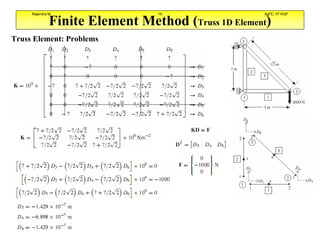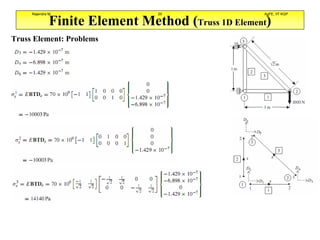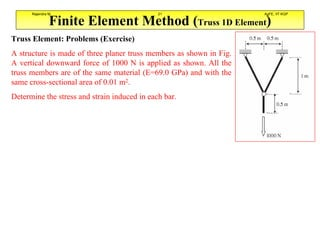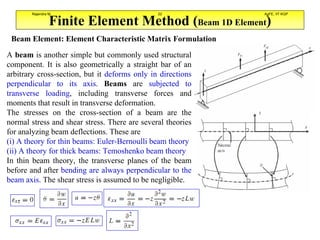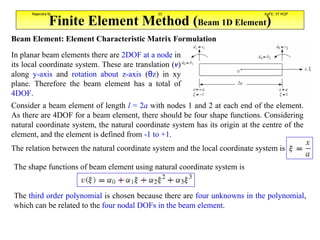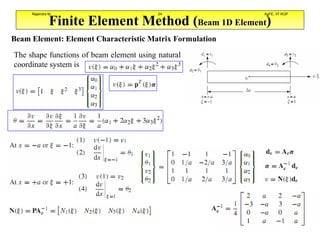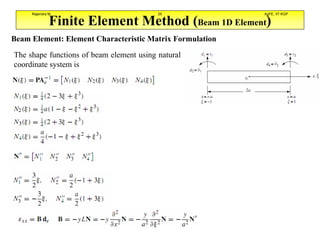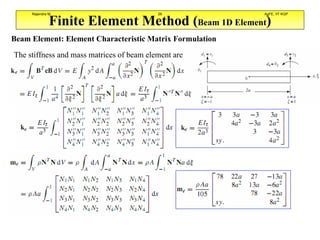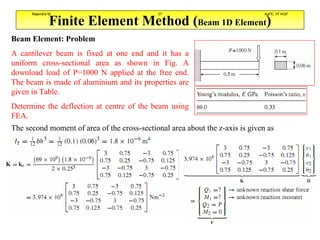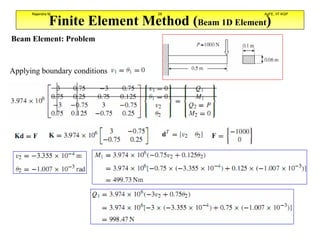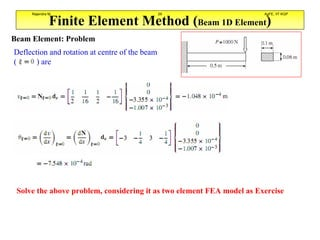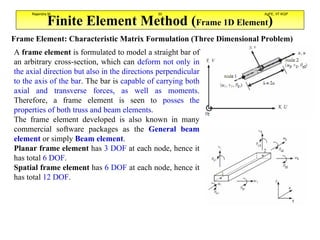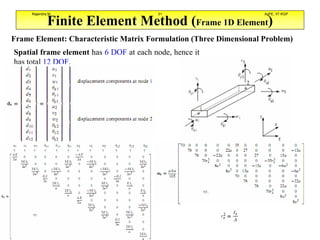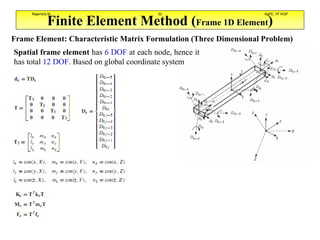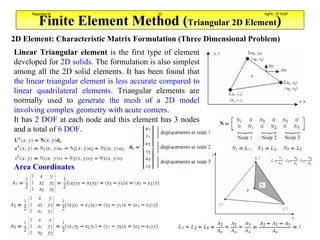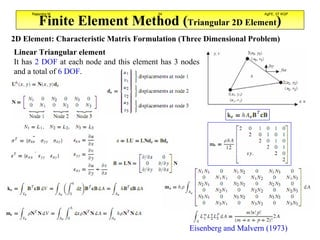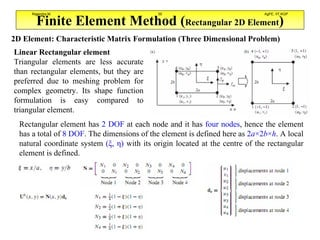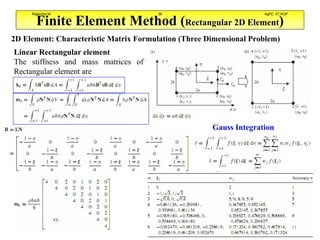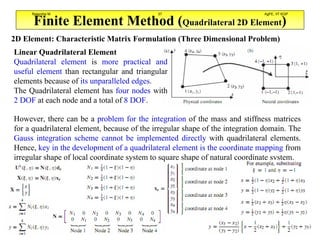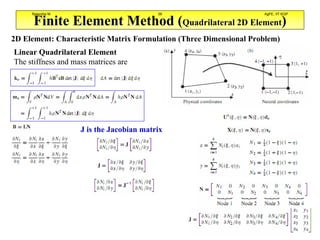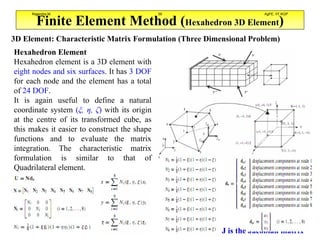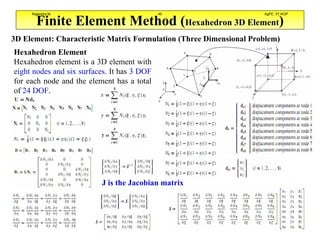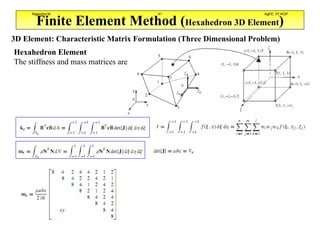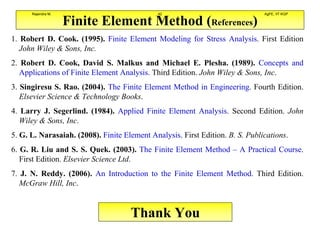1 of 42

### Finite Element Analysis Rajendra M.pdf

1. Applied Finite Element Analysis for Civil and Mechanical Engineering Applications A Conceptual Course Presented by Rajendra Machavaram Assistant Professor, AgFE, IIT Kharagpur for Faculty Development Program at Audisankara College of Engineering and Technology Gudur, Andhra Pradesh, India. Rajendra M. 1 AgFE, IIT KGP
2. Finite Element Method (Introduction & History) The Finite Element Method (FEM) is a numerical procedure for analyzing structures and continua. It was first applied to stress analysis and today it is applied to other problems, such as, heat transfer, fluid flow, lubrication, electric and magnetic fields. It is also used to design buildings, electric motors, heat engines, ships, airframes and space crafts. History: Fig. A coarse mesh of gear tooth. R. Courant (1943) described a piecewise polynomial solution for the torsion problem. The procedure was impractical at that time due to lack of digital computers. 1950s: Work in the aircraft industry introduced the FE method to practicing engineers 1960s: The name Finite Element was coined (R. W. Clough) 1963: The mathematical validity of the FE method was recognized and the method was expanded from its structural beginnings to include heat transfer, groundwater flow, magnetic fields and other areas 1970s: Large general purpose FE software began to appear 1980s: The software was available on microcomputers, complete with color graphics and pre and post processors (G. E. Smith) 1990 to Present: Application of FE using various softwares on different areas is started Rajendra M. 2 AgFE, IIT KGP
3. Finite Element Method (General Procedure) Step 1: Divide the structure or continuum into finite elements, called as mesh generation. Step 2: Formulate the properties of each element Step 3: Assemble the elements to obtain the finite element model of the structure Step 4: Apply the known loads (Initial conditions) Step 5: Apply the support conditions (Boundary conditions) Step 6: Solve the simultaneous linear algebraic equations to determine nodal DOF (Displacements/ temperatures). Step 7: Calculate the element properties, such as strains, stresses, heat flux etc. Rajendra M. 3 AgFE, IIT KGP
4. Finite Element Method (Terminology) Elements: Finite elements are fragments of the structure, these are triangular or quadrilateral areas on two dimensional space (OR) Tetrahedron or Hexahedron on three dimensional volumes. Nodes: Nodes are the connectors that fasten elements together, which appear on element boundaries. Degrees of Freedom (DOF): Number of independent parameters Shape Function: It is a polynomial function which defines the element field variables in terms of field variables of the nodes. Stiffness Matrix: It is an element characteristic matrix in structural mechanics. It relates nodal displacements to nodal forces. Conductivity Matrix: It is an element characteristic matrix in heat conduction. It relates nodal temperatures to nodal fluxes. Mass Matrix: It is an element characteristic matrix in structural dynamics. It relates nodal velocities to nodal fluxes. Rajendra M. 4 AgFE, IIT KGP
5. Finite Element Method (Problem Formulation) Finite Element Method Problem Formulation describes the procedure for determining the element characteristic matrix. There are three important ways to derive an element characteristic matrix. 1. Direct Method: It is based on physical reasoning. It is limited to very simple elements. 2. Variational Method: It is applicable to problems that can be stated by certain integral expressions such as the expression for potential energy. 3. Weighted Residual Methods: These are particularly suited to problems for which differential equations are known but no variational statement is available. a) Collocation Method: Impulse functions are selected as weighted functions. b) Subdomain Method: Each weighting function is selected as unity over a specific region. c) Galerkin’s Method: This uses the same functions for weights, that were used in the approximating equation. This approach is the basis of the finite element method for problems involving first-derivative terms (Potential or Kinetic Energy variation). d) Least Squares Method: It utilizes the residual as the weighting function and obtains a new error term. This error is minimized with respect to the unknown coefficients in the approximate solution. Rajendra M. 5 AgFE, IIT KGP
6. Finite Element Method (Problem Formulation) Constitutive Matrix Formulation (Three Dimensional Problem)–Classical Mechanics Consider a continuous three-dimensional (3D) elastic solid with a volume V and a surface area S. The solid can be loaded by body forces fb and surface forces fs in any distributed fashion in the volume of the solid. Since cij=cji, there are altogether 21 independent material constants for a fully anisotropic material Rajendra M. 6 AgFE, IIT KGP
7. Finite Element Method (Problem Formulation) Constitutive Matrix Formulation (Three Dimensional Problem)–Classical Mechanics Isotropic Material Isotropic Material – Plane Stress (Thin Shells) and Plane Strain (Thick Shells) Rajendra M. 7 AgFE, IIT KGP
8. Finite Element Method (Problem Formulation) Shape Function Formulation (Three Dimensional Problem) Consider an element with nd nodes at xi (i=1,2,..,nd), where xT = {x, y, z} for three dimensional problem. There should be nd shape functions for each displacement component for an element. Where uh is the approximation of the displacement component, pi(x) is the basis function of monomials in the space coordinates x, and αi is the coefficient for the monomial pi(x). Rajendra M. 8 AgFE, IIT KGP
9. Finite Element Method (Problem Formulation) Stiffness and Mass Matrix Formulation (Three Dimensional Problem) Strain Energy Kinetic Energy Work Done Dynamic Equilibrium Equation Static Equilibrium Equation Rajendra M. 9 AgFE, IIT KGP
10. Finite Element Method (Elements) Basic Element Shapes: The shapes, sizes, number and configuration of the elements have to be chosen carefully such that the original body or domain is simulated as closely as possible without increasing the computational effort needed for the solution. Mostly the choice of the type of element is dictated by the geometry of the body and the number of independent coordinates (DOF) necessary to describe the system. One Dimensional Elements (a) Bar (2 DOF at 2 nodes) and (b) Beam (4 DOF at 2 nodes) Two Dimensional Elements (a) Triangle (b) Rectangle (c) Quadrilateral (d) Parallelogram Three Dimensional Elements (a) Tetrahedron (b) Rectangular Prism (c) Hexahedron Rajendra M. 10 AgFE, IIT KGP
11. Finite Element Method (Bar 1D Element) Bar Element: Stiffness Matrix Formulation: Consider a uniform prismatic elastic bar of length ‘L’ with elastic modulus ‘E’ and cross-sectional area ‘A’. A node is located at each end with axially directed displacements. Direct Method: The stretch in the bar is given by And the forces is calculated as For displacement at node 1 due to force at node 2, and the displacement at node 2 due to force at node 1 is given by Rajendra M. 11 AgFE, IIT KGP
12. Finite Element Method (Bar 1D Element) Bar Element: Stiffness Matrix Formulation: Consider a uniform prismatic elastic bar of length ‘L’ with elastic modulus ‘E’ and cross-sectional area ‘A’. A node is located at each end with axially directed displacements. Variational Approach: Stiffness matrix of a element is given by Where B is the strain-displacement matrix, E is the material property matrix (Constitutive matrix) and dV is an increment of the element volume V. Rajendra M. 12 AgFE, IIT KGP
13. Finite Element Method (Truss 1D Element) The displacement within the element varies linearly, hence it is called linear element Truss Element: Stiffness Matrix Formulation: A truss is one of the simplest and most widely used structural members. It is a straight bar that is designed to take only axial forces, therefore it deforms only in its axial direction. It has one DOF at each node. Rajendra M. 13 AgFE, IIT KGP
14. Finite Element Method (Truss 1D Element) Truss Element: Stiffness Matrix Formulation: A truss is one of the simplest and most widely used structural members. It is a straight bar that is designed to take only axial forces, therefore it deforms only in its axial direction. Rajendra M. 14 AgFE, IIT KGP
15. Finite Element Method (Truss 1D Element) Truss Element: Stiffness Matrix Formulation: Considering de as element displacements in local coordinate system and De as element displacements in global coordinate system. If T is transformation matrix to transform the local coordinates to global coordinate system Rajendra M. 15 AgFE, IIT KGP
16. Finite Element Method (Truss 1D Element) Truss Element: Problems A structure is made of three planer truss members as shown in Fig. A vertical downward force of 1000 N is applied at node 2. The properties of each member is given in Table. Rajendra M. 16 AgFE, IIT KGP
17. Finite Element Method (Truss 1D Element) Truss Element: Problems Rajendra M. 17 AgFE, IIT KGP
18. Finite Element Method (Truss 1D Element) Truss Element: Problems Rajendra M. 18 AgFE, IIT KGP
19. Finite Element Method (Truss 1D Element) Truss Element: Problems Rajendra M. 19 AgFE, IIT KGP
20. Finite Element Method (Truss 1D Element) Truss Element: Problems Rajendra M. 20 AgFE, IIT KGP
21. Finite Element Method (Truss 1D Element) Truss Element: Problems (Exercise) A structure is made of three planer truss members as shown in Fig. A vertical downward force of 1000 N is applied as shown. All the truss members are of the same material (E=69.0 GPa) and with the same cross-sectional area of 0.01 m2. Determine the stress and strain induced in each bar. Rajendra M. 21 AgFE, IIT KGP
22. Finite Element Method (Beam 1D Element) Beam Element: Element Characteristic Matrix Formulation A beam is another simple but commonly used structural component. It is also geometrically a straight bar of an arbitrary cross-section, but it deforms only in directions perpendicular to its axis. Beams are subjected to transverse loading, including transverse forces and moments that result in transverse deformation. The stresses on the cross-section of a beam are the normal stress and shear stress. There are several theories for analyzing beam deflections. These are (i) A theory for thin beams: Euler-Bernoulli beam theory (ii) A theory for thick beams: Temoshenko beam theory In thin beam theory, the transverse planes of the beam before and after bending are always perpendicular to the beam axis. The shear stress is assumed to be negligible. Rajendra M. 22 AgFE, IIT KGP
23. Finite Element Method (Beam 1D Element) Beam Element: Element Characteristic Matrix Formulation In planar beam elements there are 2DOF at a node in its local coordinate system. These are translation (v) along y-axis and rotation about z-axis (θz) in xy plane. Therefore the beam element has a total of 4DOF. Consider a beam element of length l = 2a with nodes 1 and 2 at each end of the element. As there are 4DOF for a beam element, there should be four shape functions. Considering natural coordinate system, the natural coordinate system has its origin at the centre of the element, and the element is defined from -1 to +1. The relation between the natural coordinate system and the local coordinate system is The shape functions of beam element using natural coordinate system is The third order polynomial is chosen because there are four unknowns in the polynomial, which can be related to the four nodal DOFs in the beam element. Rajendra M. 23 AgFE, IIT KGP
24. Finite Element Method (Beam 1D Element) Beam Element: Element Characteristic Matrix Formulation The shape functions of beam element using natural coordinate system is Rajendra M. 24 AgFE, IIT KGP
25. Finite Element Method (Beam 1D Element) Beam Element: Element Characteristic Matrix Formulation The shape functions of beam element using natural coordinate system is Rajendra M. 25 AgFE, IIT KGP
26. Finite Element Method (Beam 1D Element) Beam Element: Element Characteristic Matrix Formulation The stiffness and mass matrices of beam element are Rajendra M. 26 AgFE, IIT KGP
27. Finite Element Method (Beam 1D Element) Beam Element: Problem A cantilever beam is fixed at one end and it has a uniform cross-sectional area as shown in Fig. A download load of P=1000 N applied at the free end. The beam is made of aluminium and its properties are given in Table. Determine the deflection at centre of the beam using FEA. The second moment of area of the cross-sectional area about the z-axis is given as Rajendra M. 27 AgFE, IIT KGP
28. Finite Element Method (Beam 1D Element) Beam Element: Problem Applying boundary conditions Rajendra M. 28 AgFE, IIT KGP
29. Finite Element Method (Beam 1D Element) Beam Element: Problem Deflection and rotation at centre of the beam ( ) are Solve the above problem, considering it as two element FEA model as Exercise Rajendra M. 29 AgFE, IIT KGP
30. Finite Element Method (Frame 1D Element) Frame Element: Characteristic Matrix Formulation (Three Dimensional Problem) A frame element is formulated to model a straight bar of an arbitrary cross-section, which can deform not only in the axial direction but also in the directions perpendicular to the axis of the bar. The bar is capable of carrying both axial and transverse forces, as well as moments. Therefore, a frame element is seen to posses the properties of both truss and beam elements. The frame element developed is also known in many commercial software packages as the General beam element or simply Beam element. Planar frame element has 3 DOF at each node, hence it has total 6 DOF. Spatial frame element has 6 DOF at each node, hence it has total 12 DOF. Rajendra M. 30 AgFE, IIT KGP
31. Finite Element Method (Frame 1D Element) Frame Element: Characteristic Matrix Formulation (Three Dimensional Problem) Spatial frame element has 6 DOF at each node, hence it has total 12 DOF. Rajendra M. 31 AgFE, IIT KGP
32. Finite Element Method (Frame 1D Element) Frame Element: Characteristic Matrix Formulation (Three Dimensional Problem) Spatial frame element has 6 DOF at each node, hence it has total 12 DOF. Based on global coordinate system Rajendra M. 32 AgFE, IIT KGP
33. Finite Element Method (Triangular 2D Element) 2D Element: Characteristic Matrix Formulation (Three Dimensional Problem) Linear Triangular element is the first type of element developed for 2D solids. The formulation is also simplest among all the 2D solid elements. It has been found that the linear triangular element is less accurate compared to linear quadrilateral elements. Triangular elements are normally used to generate the mesh of a 2D model involving complex geometry with acute corners. It has 2 DOF at each node and this element has 3 nodes and a total of 6 DOF. Area Coordinates Rajendra M. 33 AgFE, IIT KGP
34. Finite Element Method (Triangular 2D Element) 2D Element: Characteristic Matrix Formulation (Three Dimensional Problem) Linear Triangular element It has 2 DOF at each node and this element has 3 nodes and a total of 6 DOF. Eisenberg and Malvern (1973) Rajendra M. 34 AgFE, IIT KGP
35. Finite Element Method (Rectangular 2D Element) 2D Element: Characteristic Matrix Formulation (Three Dimensional Problem) Linear Rectangular element Triangular elements are less accurate than rectangular elements, but they are preferred due to meshing problem for complex geometry. Its shape function formulation is easy compared to triangular element. Rectangular element has 2 DOF at each node and it has four nodes, hence the element has a total of 8 DOF. The dimensions of the element is defined here as 2a×2b×h. A local natural coordinate system (ξ, η) with its origin located at the centre of the rectangular element is defined. Rajendra M. 35 AgFE, IIT KGP
36. Finite Element Method (Rectangular 2D Element) 2D Element: Characteristic Matrix Formulation (Three Dimensional Problem) Linear Rectangular element The stiffness and mass matrices of Rectangular element are Gauss Integration Rajendra M. 36 AgFE, IIT KGP
37. Finite Element Method (Quadrilateral 2D Element) 2D Element: Characteristic Matrix Formulation (Three Dimensional Problem) Linear Quadrilateral Element Quadrilateral element is more practical and useful element than rectangular and triangular elements because of its unparalleled edges. The Quadrilateral element has four nodes with 2 DOF at each node and a total of 8 DOF. However, there can be a problem for the integration of the mass and stiffness matrices for a quadrilateral element, because of the irregular shape of the integration domain. The Gauss integration scheme cannot be implemented directly with quadrilateral elements. Hence, key in the development of a quadrilateral element is the coordinate mapping from irregular shape of local coordinate system to square shape of natural coordinate system. Rajendra M. 37 AgFE, IIT KGP
38. Finite Element Method (Quadrilateral 2D Element) 2D Element: Characteristic Matrix Formulation (Three Dimensional Problem) Linear Quadrilateral Element The stiffness and mass matrices are J is the Jacobian matrix Rajendra M. 38 AgFE, IIT KGP
39. Finite Element Method (Hexahedron 3D Element) 3D Element: Characteristic Matrix Formulation (Three Dimensional Problem) Hexahedron Element Hexahedron element is a 3D element with eight nodes and six surfaces. It has 3 DOF for each node and the element has a total of 24 DOF. It is again useful to define a natural coordinate system (ξ, η, ζ) with its origin at the centre of its transformed cube, as this makes it easier to construct the shape functions and to evaluate the matrix integration. The characteristic matrix formulation is similar to that of Quadrilateral element. J is the Jacobian matrix Rajendra M. 39 AgFE, IIT KGP
40. Finite Element Method (Hexahedron 3D Element) 3D Element: Characteristic Matrix Formulation (Three Dimensional Problem) Hexahedron Element Hexahedron element is a 3D element with eight nodes and six surfaces. It has 3 DOF for each node and the element has a total of 24 DOF. J is the Jacobian matrix Rajendra M. 40 AgFE, IIT KGP
41. Finite Element Method (Hexahedron 3D Element) 3D Element: Characteristic Matrix Formulation (Three Dimensional Problem) Hexahedron Element The stiffness and mass matrices are Rajendra M. 41 AgFE, IIT KGP
42. Finite Element Method (References) 1. Robert D. Cook. (1995). Finite Element Modeling for Stress Analysis. First Edition John Wiley & Sons, Inc. 2. Robert D. Cook, David S. Malkus and Michael E. Plesha. (1989). Concepts and Applications of Finite Element Analysis. Third Edition. John Wiley & Sons, Inc. 3. Singiresu S. Rao. (2004). The Finite Element Method in Engineering. Fourth Edition. Elsevier Science & Technology Books. 4. Larry J. Segerlind. (1984). Applied Finite Element Analysis. Second Edition. John Wiley & Sons, Inc. 5. G. L. Narasaiah. (2008). Finite Element Analysis. First Edition. B. S. Publications. 6. G. R. Liu and S. S. Quek. (2003). The Finite Element Method – A Practical Course. First Edition. Elsevier Science Ltd. 7. J. N. Reddy. (2006). An Introduction to the Finite Element Method. Third Edition. McGraw Hill, Inc. Thank You Rajendra M. 42 AgFE, IIT KGP